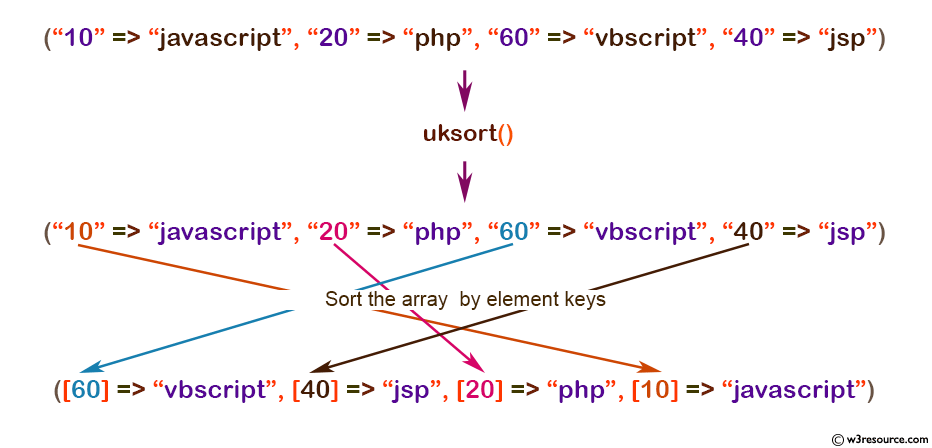# PHP: uksort() function

## PHP: Sort an array by keys using a user-defined comparison function

The uksort() function is used to sort an array by the element keys using a user-defined comparison function. If the array you wish to sort needs to be sorted by some non-trivial criteria, you should use this function.

Note: If two members compare as equal, their relative order in the sorted array is undefined.

Version:

(PHP 4 and above)

Syntax:

`uksort(array_name, user_defined_function)`

Parameters:

Name Description Required /
Optional
Type
array_name The input array. Required Array
user_defined_function User supplied function. Required
-

Return value

TRUE on success or FALSE on failure.

Value Type : Boolean.

Example:

``````<?php
function my_sort(\$x, \$y)
{
if (\$x == \$y) return 0;
return (\$x > \$y) ? -1 : 1;
}
\$people = array("10" => "javascript",
"20" => "php", "60" => "vbscript",
"40" => "jsp");
uksort(\$people, "my_sort");
print_r (\$people);
?>
``````

Output:

`Array ( => vbscript  => jsp  => php  => javascript )`

Pictorial Presentation:View the example in the browser

Practice here online:

PHP Function Reference

Previous: uasort
Next: usort

﻿

## PHP: Tips of the Day

Filters the collection using the given callback

Example:

```<?php
function tips_reject(\$items, \$func)
{
return array_values(array_diff(\$items, array_filter(\$items, \$func)));
}

print_r(tips_reject(['Apple', 'Pear', 'Kiwi', 'Banana'], function (\$item) {
return strlen(\$item) > 4;
}));
?>
```

Output:

```Array
(
 => Pear
 => Kiwi
)
```